# 8086-instruction-set-ppt

26. Nov 2014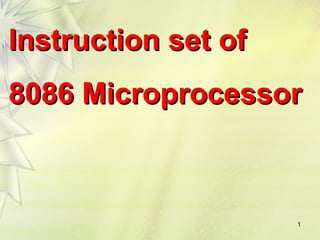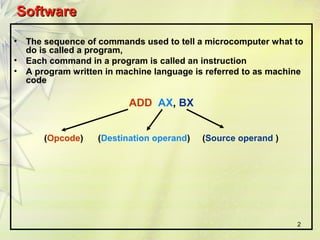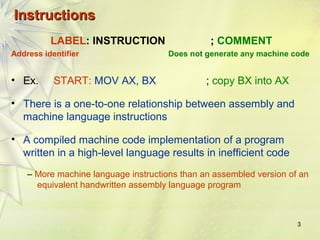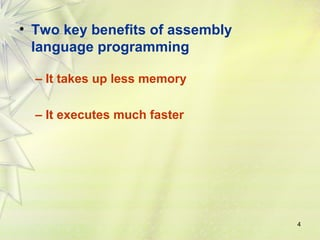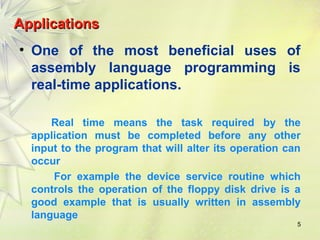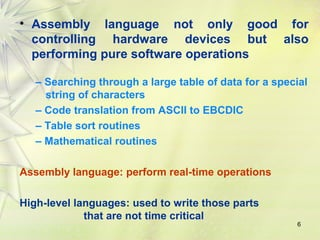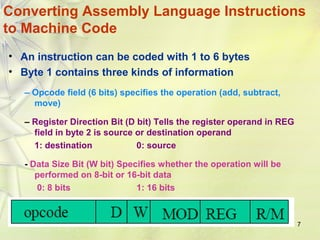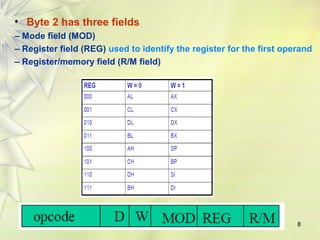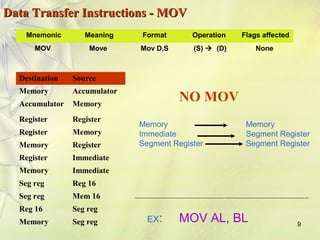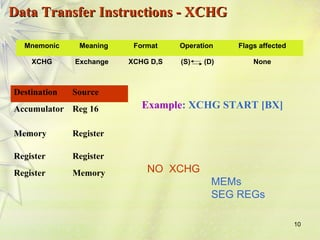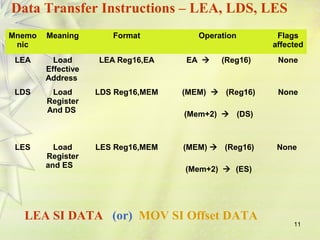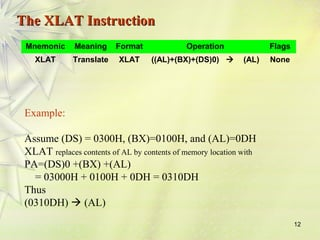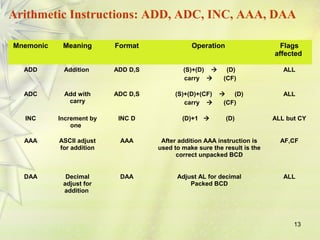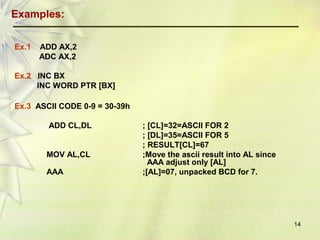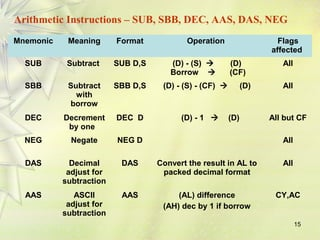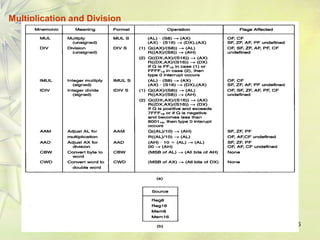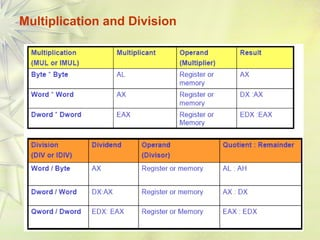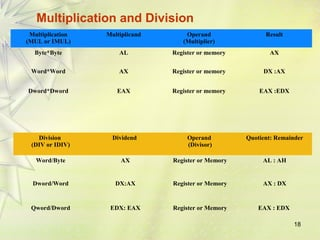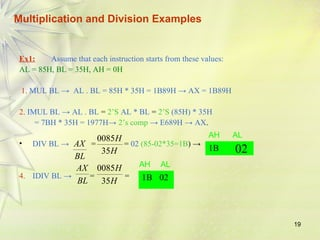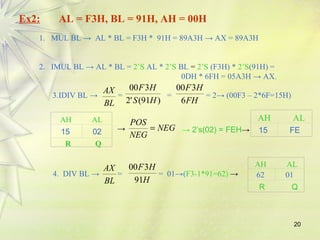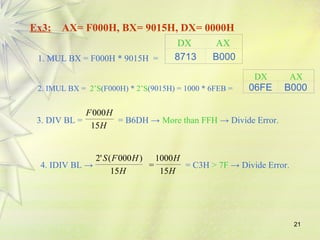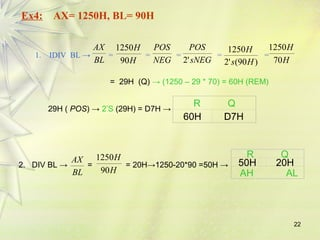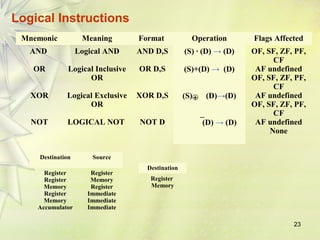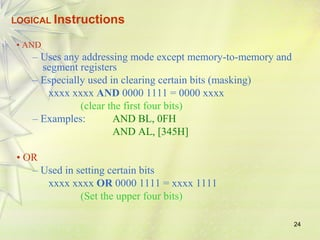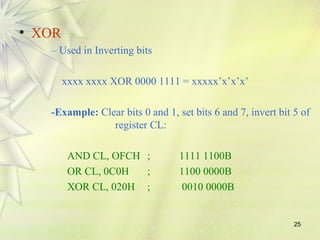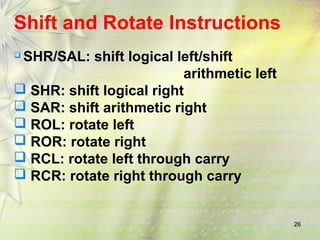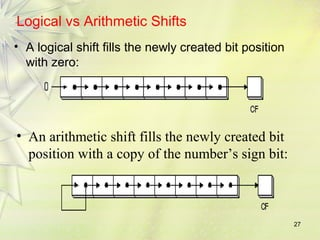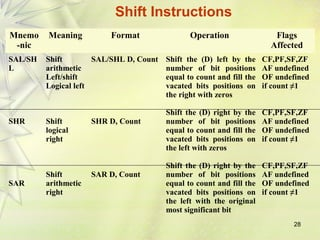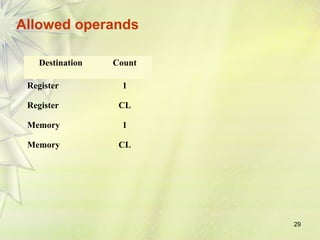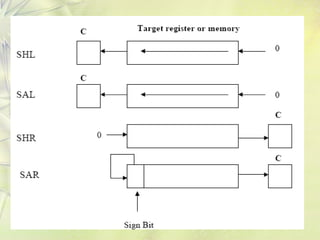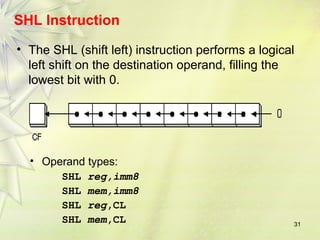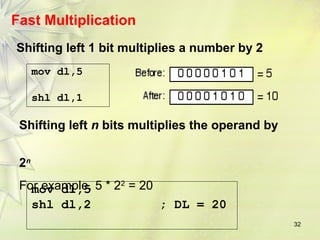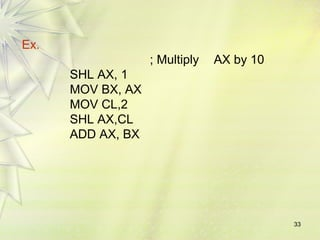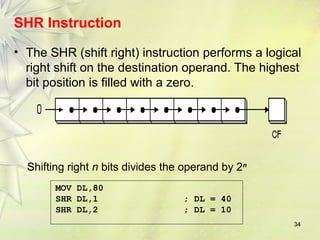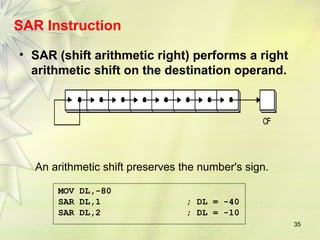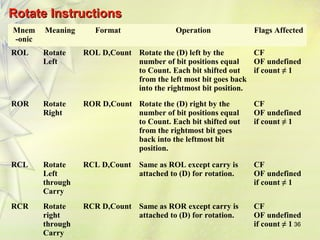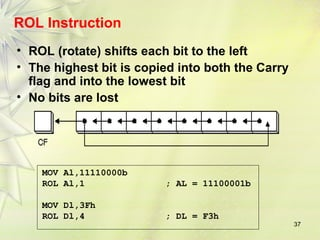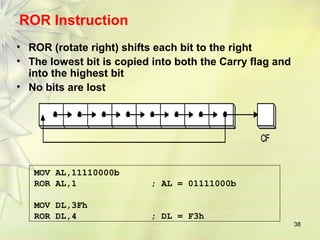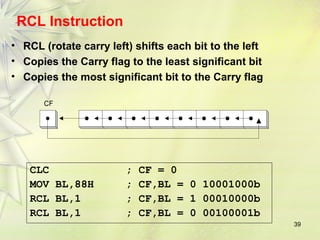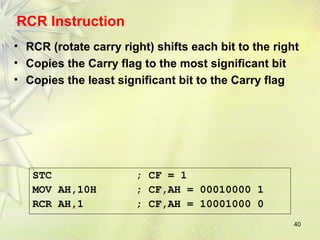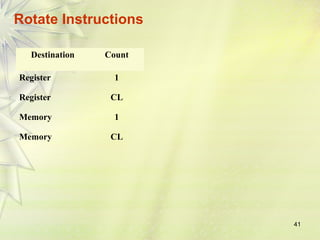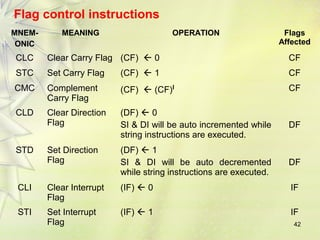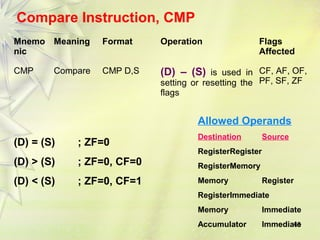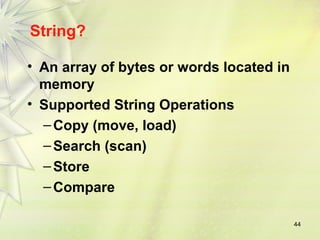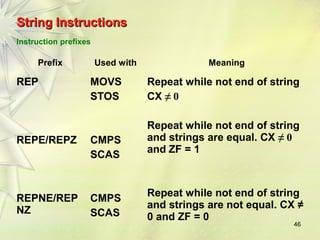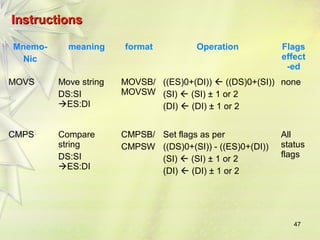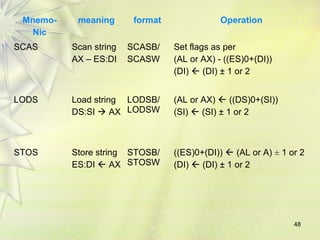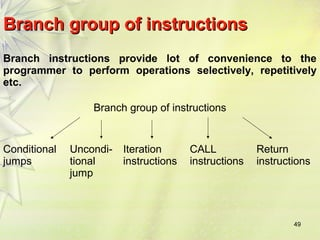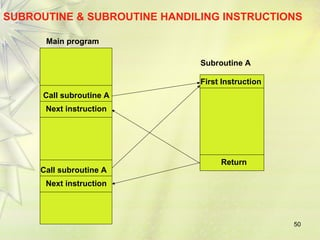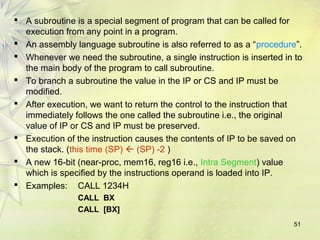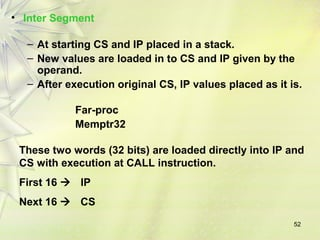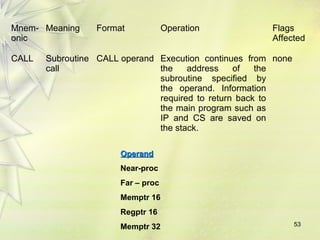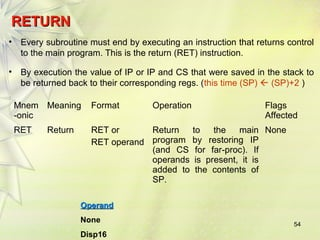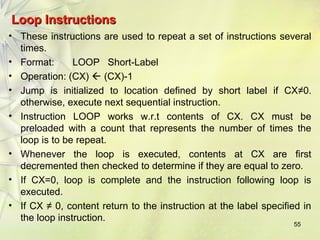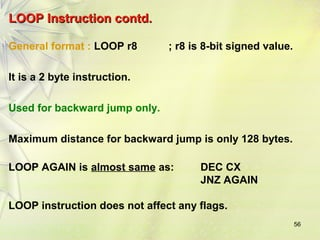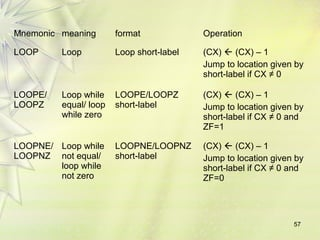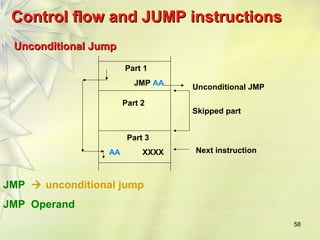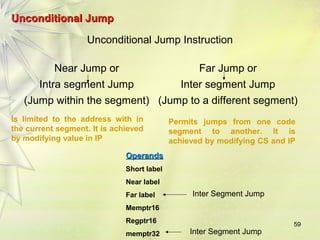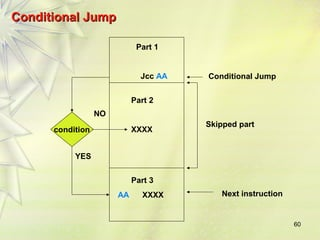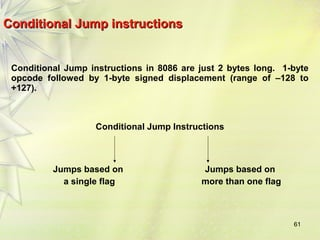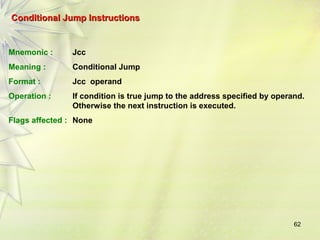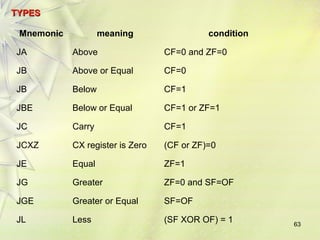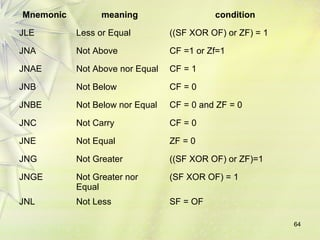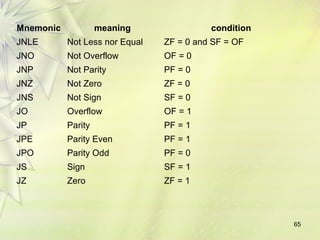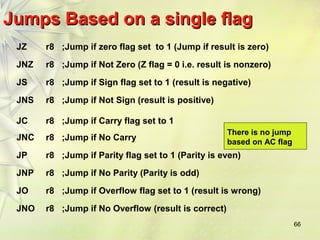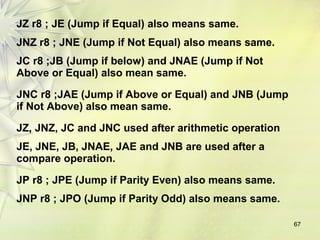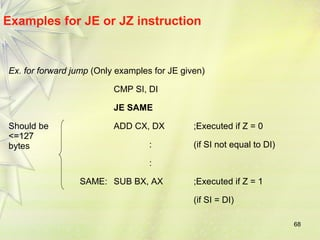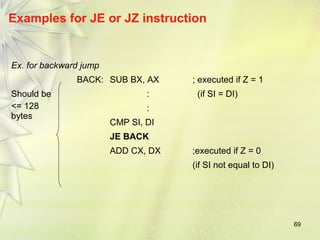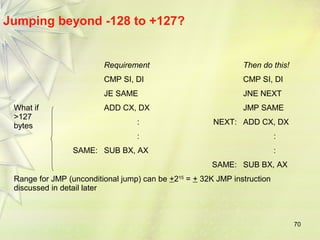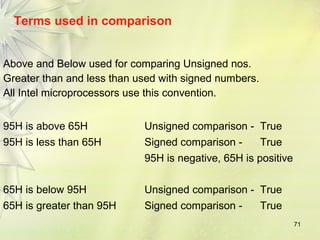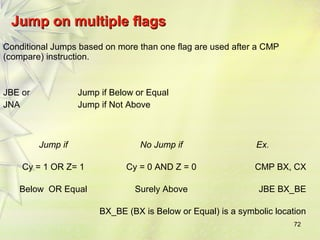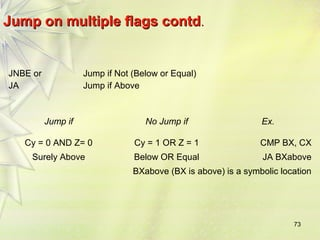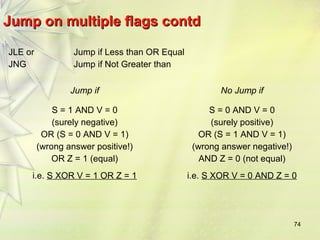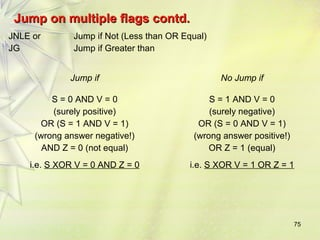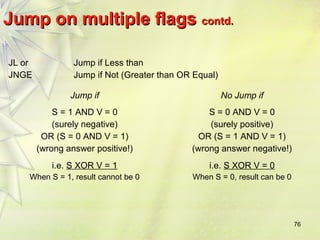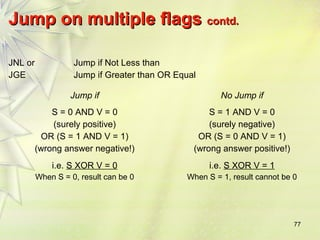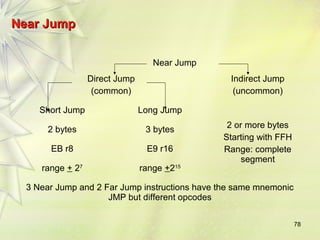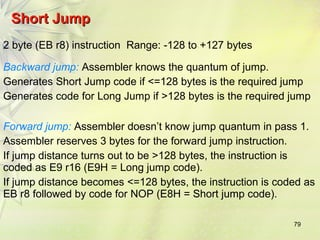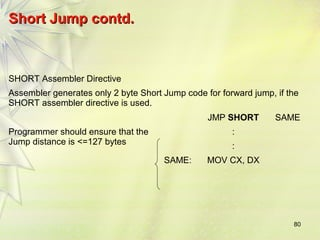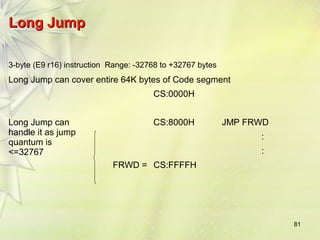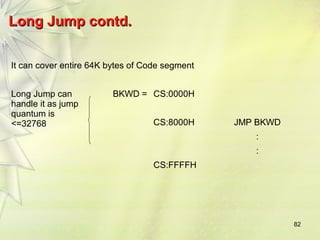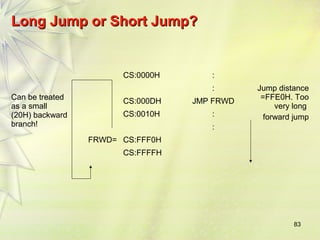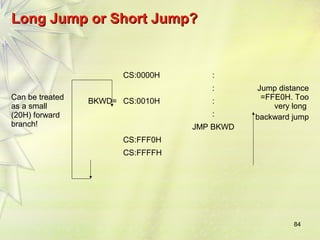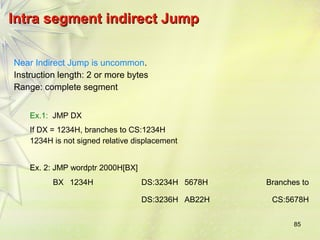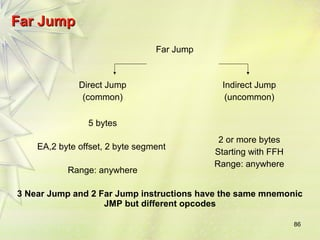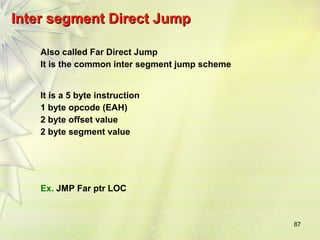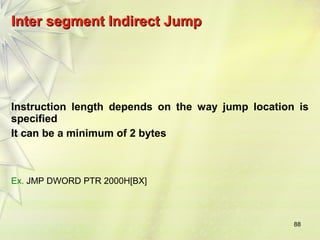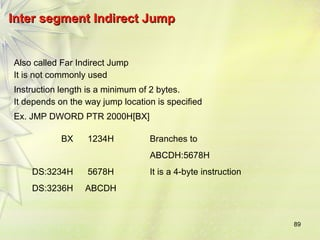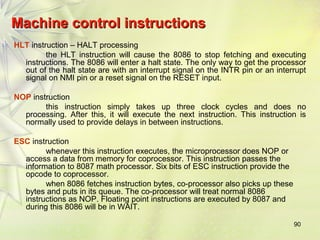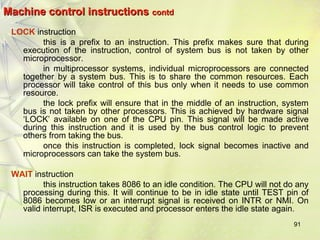1 von 91

### 8086-instruction-set-ppt

• 1. IInnssttrruuccttiioonn sseett ooff 88008866 MMiiccrroopprroocceessssoorr 1
• 2. SSooffttwwaarree • The sequence of commands used to tell a microcomputer what to do is called a program, • Each command in a program is called an instruction • A program written in machine language is referred to as machine code 2 ADD AX, BX (Opcode) (Destination operand) (Source operand )
• 3. 3 IInnssttrruuccttiioonnss LABEL: INSTRUCTION ; COMMENT Address identifier Does not generate any machine code • Ex. START: MOV AX, BX ; copy BX into AX • There is a one-to-one relationship between assembly and machine language instructions • A compiled machine code implementation of a program written in a high-level language results in inefficient code – More machine language instructions than an assembled version of an equivalent handwritten assembly language program
• 4. 4 • Two key benefits of assembly language programming – It takes up less memory – It executes much faster
• 5. AApppplliiccaattiioonnss • One of the most beneficial uses of assembly language programming is real-time applications. Real time means the task required by the application must be completed before any other input to the program that will alter its operation can occur For example the device service routine which controls the operation of the floppy disk drive is a good example that is usually written in assembly language 5
• 6. • Assembly language not only good for controlling hardware devices but also performing pure software operations – Searching through a large table of data for a special string of characters – Code translation from ASCII to EBCDIC – Table sort routines – Mathematical routines 6 Assembly language: perform real-time operations High-level languages: used to write those parts that are not time critical
• 7. Converting Assembly Language Instructions to Machine Code • An instruction can be coded with 1 to 6 bytes • Byte 1 contains three kinds of information 7 – Opcode field (6 bits) specifies the operation (add, subtract, move) – Register Direction Bit (D bit) Tells the register operand in REG field in byte 2 is source or destination operand 1: destination 0: source - Data Size Bit (W bit) Specifies whether the operation will be performed on 8-bit or 16-bit data 0: 8 bits 1: 16 bits
• 8. • Byte 2 has three fields – Mode field (MOD) – Register field (REG) used to identify the register for the first operand – Register/memory field (R/M field) 8
• 9. 9 DDaattaa TTrraannssffeerr IInnssttrruuccttiioonnss -- MMOOVV Mnemonic Meaning Format Operation Flags affected MOV Move Mov D,S (S)  (D) None Destination Source Memory Accumulator Accumulator Memory Register Register Register Memory Memory Register Register Immediate Memory Immediate Seg reg Reg 16 Seg reg Mem 16 Reg 16 Seg reg Memory Seg reg NO MOV Memory Immediate Segment Register Memory Segment Register Segment Register EX: MOV AL, BL
• 10. 10 Data TTrraannssffeerr IInnssttrruuccttiioonnss -- XXCCHHGG Mnemonic Meaning Format Operation Flags affected XCHG Exchange XCHG D,S (S) (D) None Destination Source Accumulator Reg 16 Memory Register Register Register Register Memory Example: XCHG START [BX] NO XCHG MEMs SEG REGs
• 11. 11 Data Transfer Instructions – LEA, LDS, LES Mnemo nic Meaning Format Operation Flags affected LEA Load Effective Address LEA Reg16,EA EA  (Reg16) None LDS Load Register And DS LDS Reg16,MEM (MEM)  (Reg16) (Mem+2)  (DS) None LES Load Register and ES LES Reg16,MEM (MEM)  (Reg16) (Mem+2)  (ES) None LEA SI DATA (or) MOV SI Offset DATA
• 12. 12 TThhee XXLLAATT IInnssttrruuccttiioonn Mnemonic Meaning Format Operation Flags XLAT Translate XLAT ((AL)+(BX)+(DS)0)  (AL) None Example: Assume (DS) = 0300H, (BX)=0100H, and (AL)=0DH XLAT replaces contents of AL by contents of memory location with PA=(DS)0 +(BX) +(AL) = 03000H + 0100H + 0DH = 0310DH Thus (0310DH)  (AL)
• 14. 14 Examples: Ex.1 ADD AX,2 ADC AX,2 Ex.2 INC BX INC WORD PTR [BX] Ex.3 ASCII CODE 0-9 = 30-39h ADD CL,DL ; [CL]=32=ASCII FOR 2 ; [DL]=35=ASCII FOR 5 ; RESULT[CL]=67 MOV AL,CL ;Move the ascii result into AL since AAA adjust only [AL] AAA ;[AL]=07, unpacked BCD for 7.
• 15. Arithmetic Instructions – SUB, SBB, DEC, AAS, DAS, NEG Mnemonic Meaning Format Operation Flags affected 15 SUB Subtract SUB D,S (D) - (S)  (D) Borrow  (CF) All SBB Subtract with borrow SBB D,S (D) - (S) - (CF)  (D) All DEC Decrement by one DEC D (D) - 1  (D) All but CF NEG Negate NEG D All DAS Decimal adjust for subtraction DAS Convert the result in AL to packed decimal format All AAS ASCII adjust for subtraction AAS (AL) difference (AH) dec by 1 if borrow CY,AC
• 18. 18 Multiplication and Division Multiplication (MUL or IMUL) Multiplicand Operand (Multiplier) Result Byte*Byte AL Register or memory AX Word*Word AX Register or memory DX :AX Dword*Dword EAX Register or memory EAX :EDX Division (DIV or IDIV) Dividend Operand (Divisor) Quotient: Remainder Word/Byte AX Register or Memory AL : AH Dword/Word DX:AX Register or Memory AX : DX Qword/Dword EDX: EAX Register or Memory EAX : EDX
• 19. 19 Multiplication and Division Examples Ex1: Assume that each instruction starts from these values: AL = 85H, BL = 35H, AH = 0H 1. MUL BL → AL . BL = 85H * 35H = 1B89H → AX = 1B89H 2. IMUL BL → AL . BL = 2’S AL * BL = 2’S (85H) * 35H = 7BH * 35H = 1977H→ 2’s comp → E689H → AX. H 0085 AX • DIV BL → = = 02 (85-02*35=1B) → AH AL H 1B 35 AX H 0085 4. IDIV BL → = = 02 BL BL H 35 AH AL 1B 02
• 20. 00 3 AH AL 20 Ex2: AL = F3H, BL = 91H, AH = 00H 1. MUL BL → AL * BL = F3H * 91H = 89A3H → AX = 89A3H 2. IMUL BL → AL * BL = 2’S AL * 2’S BL = 2’S (F3H) * 2’S(91H) = 0DH * 6FH = 05A3H → AX. AX F H 00 3 S H F H 6 00 3 3.IDIV BL → = = = 2→ (00F3 – 2*6F=15H) BL 2' (91 ) FH AH AL 15 02 R Q POS = NEG → 2’s(02) = FEH→ NEG AH AL → 15 FE AX F H 91 4. DIV BL → = = 01→(F3-1*91=62) → BL H 62 01 R Q
• 21. 21 Ex3: AX= F000H, BX= 9015H, DX= 0000H 1. MUL BX = F000H * 9015H = DX AX 8713 B000 2. IMUL BX = 2’S(F000H) * 2’S(9015H) = 1000 * 6FEB = DX AX 06FE B000 F 000 H 15 3. DIV BL = = B6DH → More than H FFH → Divide Error. S F H 15 2' ( 000 ) H 1000 4. IDIV BL → H = H = C3H > 7F → Divide Error. 15
• 22. 22 Ex4: AX= 1250H, BL= 90H AX H 1250 POS POS H 2' 2' (90 ) 1250 1. IDIV BL → = = = = BL = H 90 NEG sNEG 1250 s H H H 70 = 29H (Q) → (1250 – 29 * 70) = 60H (REM) 29H ( POS) → 2’S (29H) = D7H → R Q 60H D7H AX 1250 H R Q 2. DIV BL → = = 20H→1250-20*90 =50H → BL H 90 50H 20H AH AL
• 23. Mnemonic Meaning Format Operation Flags Affected AND 23 OR XOR NOT Logical AND Logical Inclusive OR Logical Exclusive OR LOGICAL NOT AND D,S OR D,S XOR D,S NOT D (S) · (D) → (D) (S)+(D) → (D) (S) (D)→(D) _ (D) → (D) OF, SF, ZF, PF, CF AF undefined OF, SF, ZF, PF, CF AF undefined OF, SF, ZF, PF, CF AF undefined None + Logical Instructions Destination Source Register Register Memory Register Memory Accumulator Register Memory Register Immediate Immediate Immediate Destination Register Memory
• 24. 24 LOGICAL Instructions • AND – Uses any addressing mode except memory-to-memory and segment registers – Especially used in clearing certain bits (masking) xxxx xxxx AND 0000 1111 = 0000 xxxx (clear the first four bits) – Examples: AND BL, 0FH AND AL, [345H] • OR – Used in setting certain bits xxxx xxxx OR 0000 1111 = xxxx 1111 (Set the upper four bits)
• 25. 25 • XOR – Used in Inverting bits xxxx xxxx XOR 0000 1111 = xxxxx’x’x’x’ -Example: Clear bits 0 and 1, set bits 6 and 7, invert bit 5 of register CL: AND CL, OFCH ; 1111 1100B OR CL, 0C0H ; 1100 0000B XOR CL, 020H ; 0010 0000B
• 26. 26 Shift and Rotate Instructions  SHR/SAL: shift logical left/shift arithmetic left  SHR: shift logical right  SAR: shift arithmetic right  ROL: rotate left  ROR: rotate right  RCL: rotate left through carry  RCR: rotate right through carry
• 27. 27 Logical vs Arithmetic Shifts • A logical shift fills the newly created bit position with zero: • An arithmetic shift fills the newly created bit position with a copy of the number’s sign bit:
• 28. 28 Mnemo -nic Meaning Format Operation Flags Affected SAL/SH L SHR SAR Shift arithmetic Left/shift Logical left Shift logical right Shift arithmetic right SAL/SHL D, Count SHR D, Count SAR D, Count Shift the (D) left by the number of bit positions equal to count and fill the vacated bits positions on the right with zeros Shift the (D) right by the number of bit positions equal to count and fill the vacated bits positions on the left with zeros Shift the (D) right by the number of bit positions equal to count and fill the vacated bits positions on the left with the original most significant bit CF,PF,SF,ZF AF undefined OF undefined if count ≠1 CF,PF,SF,ZF AF undefined OF undefined if count ≠1 CF,PF,SF,ZF AF undefined OF undefined if count ≠1 Shift Instructions
• 29. 29 Allowed operands Destination Count Register 1 Register CL Memory 1 Memory CL
• 30. 30
• 31. SHL Instruction • The SHL (shift left) instruction performs a logical left shift on the destination operand, filling the lowest bit with 0. 31 • Operand types: SHL reg,imm8 SHL mem,imm8 SHL reg,CL SHL mem,CL
• 32. 32 Fast Multiplication Shifting left 1 bit multiplies a number by 2 mov dl,5 shl dl,1 Shifting left n bits multiplies the operand by 2n For example, 5 * 22 = 20 mov dl,5 shl dl,2 ; DL = 20
• 33. 33 Ex. ; Multiply AX by 10 SHL AX, 1 MOV BX, AX MOV CL,2 SHL AX,CL ADD AX, BX
• 34. SHR Instruction • The SHR (shift right) instruction performs a logical right shift on the destination operand. The highest bit position is filled with a zero. 34 Shifting right n bits divides the operand by 2n MOV DL,80 SHR DL,1 ; DL = 40 SHR DL,2 ; DL = 10
• 35. 35 SAR Instruction • SAR (shift arithmetic right) performs a right arithmetic shift on the destination operand. An arithmetic shift preserves the number's sign. MOV DL,-80 SAR DL,1 ; DL = -40 SAR DL,2 ; DL = -10
• 36. 36 RRoottaattee IInnssttrruuccttiioonnss Mnem -onic Meaning Format Operation Flags Affected ROL Rotate Left ROL D,Count Rotate the (D) left by the number of bit positions equal to Count. Each bit shifted out from the left most bit goes back into the rightmost bit position. CF OF undefined if count ≠ 1 ROR Rotate Right ROR D,Count Rotate the (D) right by the number of bit positions equal to Count. Each bit shifted out from the rightmost bit goes back into the leftmost bit position. CF OF undefined if count ≠ 1 RCL Rotate Left through Carry RCL D,Count Same as ROL except carry is attached to (D) for rotation. CF OF undefined if count ≠ 1 RCR Rotate right through Carry RCR D,Count Same as ROR except carry is attached to (D) for rotation. CF OF undefined if count ≠ 1
• 37. 37 ROL Instruction • ROL (rotate) shifts each bit to the left • The highest bit is copied into both the Carry flag and into the lowest bit • No bits are lost MOV Al,11110000b ROL Al,1 ; AL = 11100001b MOV Dl,3Fh ROL Dl,4 ; DL = F3h
• 38. 38 ROR Instruction • ROR (rotate right) shifts each bit to the right • The lowest bit is copied into both the Carry flag and into the highest bit • No bits are lost MOV AL,11110000b ROR AL,1 ; AL = 01111000b MOV DL,3Fh ROR DL,4 ; DL = F3h
• 39. 39 RCL Instruction • RCL (rotate carry left) shifts each bit to the left • Copies the Carry flag to the least significant bit • Copies the most significant bit to the Carry flag CF CLC ; CF = 0 MOV BL,88H ; CF,BL = 0 10001000b RCL BL,1 ; CF,BL = 1 00010000b RCL BL,1 ; CF,BL = 0 00100001b
• 40. RCR Instruction • RCR (rotate carry right) shifts each bit to the right • Copies the Carry flag to the most significant bit • Copies the least significant bit to the Carry flag 40 STC ; CF = 1 MOV AH,10H ; CF,AH = 00010000 1 RCR AH,1 ; CF,AH = 10001000 0
• 41. 41 Rotate Instructions Destination Count Register 1 Register CL Memory 1 Memory CL
• 42. Flag control instructions MNEM-ONIC MEANING OPERATION Flags Affected CLC Clear Carry Flag (CF)  0 CF STC Set Carry Flag (CF)  1 CF CMC Complement 42 Carry Flag (CF)  (CF)l CF CLD Clear Direction Flag (DF)  0 SI & DI will be auto incremented while string instructions are executed. DF STD Set Direction Flag (DF)  1 SI & DI will be auto decremented while string instructions are executed. DF CLI Clear Interrupt Flag (IF)  0 IF STI Set Interrupt Flag (IF)  1 IF
• 43. 43 Compare Instruction, CMP Mnemo nic Meaning Format Operation Flags Affected CMP Compare CMP D,S (D) – (S) is used in setting or resetting the flags CF, AF, OF, PF, SF, ZF (D) = (S) ; ZF=0 (D) > (S) ; ZF=0, CF=0 (D) < (S) ; ZF=0, CF=1 Allowed Operands Destination Source RegisterRegister RegisterMemory Memory Register RegisterImmediate Memory Immediate Accumulator Immediate
• 44. 44 String? • An array of bytes or words located in memory • Supported String Operations –Copy (move, load) –Search (scan) – Store –Compare
• 45. 45 String Instruction Basics • Source DS:SI, Destination ES:DI – You must ensure DS and ES are correct – You must ensure SI and DI are offsets into DS and ES respectively • Direction Flag (0 = Up, 1 = Down) – CLD - Increment addresses (left to right) – STD - Decrement addresses (right to left)
• 46. 46 SSttrriinngg IInnssttrruuccttiioonnss Instruction prefixes Prefix Used with Meaning REP REPE/REPZ REPNE/REP NZ MOVS STOS CMPS SCAS CMPS SCAS Repeat while not end of string CX ≠ 0 Repeat while not end of string and strings are equal. CX ≠ 0 and ZF = 1 Repeat while not end of string and strings are not equal. CX ≠ 0 and ZF = 0
• 47. 47 IInnssttrruuccttiioonnss Mnemo- Nic meaning format Operation Flags effect -ed MOVS Move string DS:SI ES:DI MOVSB/ MOVSW ((ES)0+(DI))  ((DS)0+(SI)) (SI)  (SI) ± 1 or 2 (DI)  (DI) ± 1 or 2 none CMPS Compare string DS:SI ES:DI CMPSB/ CMPSW Set flags as per ((DS)0+(SI)) - ((ES)0+(DI)) (SI)  (SI) ± 1 or 2 (DI)  (DI) ± 1 or 2 All status flags
• 48. 48 Mnemo- Nic meaning format Operation SCAS Scan string AX – ES:DI SCASB/ SCASW Set flags as per (AL or AX) - ((ES)0+(DI)) (DI)  (DI) ± 1 or 2 LODS Load string DS:SI  AX LODSB/ LODSW (AL or AX)  ((DS)0+(SI)) (SI)  (SI) ± 1 or 2 STOS Store string ES:DI  AX STOSB/ STOSW ((ES)0+(DI))  (AL or A) ± 1 or 2 (DI)  (DI) ± 1 or 2
• 49. BBrraanncchh ggrroouupp ooff iinnssttrruuccttiioonnss Branch instructions provide lot of convenience to the programmer to perform operations selectively, repetitively etc. 49 Branch group of instructions Conditional jumps Uncondi-tional jump Iteration instructions CALL instructions Return instructions
• 50. SUBROUTINE & SUBROUTINE HANDILING INSTRUCTIONS 50 Main program Call subroutine A Next instruction Call subroutine A Next instruction Subroutine A First Instruction Return
• 51.  A subroutine is a special segment of program that can be called for execution from any point in a program.  An assembly language subroutine is also referred to as a “procedure”.  Whenever we need the subroutine, a single instruction is inserted in to the main body of the program to call subroutine.  To branch a subroutine the value in the IP or CS and IP must be modified.  After execution, we want to return the control to the instruction that immediately follows the one called the subroutine i.e., the original value of IP or CS and IP must be preserved.  Execution of the instruction causes the contents of IP to be saved on the stack. (this time (SP)  (SP) -2 )  A new 16-bit (near-proc, mem16, reg16 i.e., Intra Segment) value which is specified by the instructions operand is loaded into IP.  Examples: CALL 1234H 51 CALL BX CALL [BX]
• 52. 52 • Inter Segment – At starting CS and IP placed in a stack. – New values are loaded in to CS and IP given by the operand. – After execution original CS, IP values placed as it is. Far-proc Memptr32 These two words (32 bits) are loaded directly into IP and CS with execution at CALL instruction. First 16  IP Next 16  CS
• 53. Mnem-onic Meaning Format Operation Flags Affected 53 CALL Subroutine call CALL operand Execution continues from the address of the subroutine specified by the operand. Information required to return back to the main program such as IP and CS are saved on the stack. none OOppeerraanndd Near-proc Far – proc Memptr 16 Regptr 16 Memptr 32
• 54. RREETTUURRNN • Every subroutine must end by executing an instruction that returns control to the main program. This is the return (RET) instruction. • By execution the value of IP or IP and CS that were saved in the stack to be returned back to their corresponding regs. (this time (SP)  (SP)+2 ) Mnem -onic 54 Meaning Format Operation Flags Affected RET Return RET or RET operand Return to the main program by restoring IP (and CS for far-proc). If operands is present, it is added to the contents of SP. None OOppeerraanndd None Disp16
• 55. LLoooopp IInnssttrruuccttiioonnss • These instructions are used to repeat a set of instructions several times. • Format: LOOP Short-Label • Operation: (CX)  (CX)-1 • Jump is initialized to location defined by short label if CX≠0. otherwise, execute next sequential instruction. • Instruction LOOP works w.r.t contents of CX. CX must be preloaded with a count that represents the number of times the loop is to be repeat. • Whenever the loop is executed, contents at CX are first decremented then checked to determine if they are equal to zero. • If CX=0, loop is complete and the instruction following loop is executed. • If CX ≠ 0, content return to the instruction at the label specified in the loop instruction. 55
• 56. 56 LLOOOOPP IInnssttrruuccttiioonn ccoonnttdd.. General format : LOOP r8 ; r8 is 8-bit signed value. It is a 2 byte instruction. Used for backward jump only. Maximum distance for backward jump is only 128 bytes. LOOP AGAIN is almost same as: DEC CX JNZ AGAIN LOOP instruction does not affect any flags.
• 57. 57 Mnemonic meaning format Operation LOOP Loop Loop short-label (CX)  (CX) – 1 Jump to location given by short-label if CX ≠ 0 LOOPE/ LOOPZ Loop while equal/ loop while zero LOOPE/LOOPZ short-label (CX)  (CX) – 1 Jump to location given by short-label if CX ≠ 0 and ZF=1 LOOPNE/ LOOPNZ Loop while not equal/ loop while not zero LOOPNE/LOOPNZ short-label (CX)  (CX) – 1 Jump to location given by short-label if CX ≠ 0 and ZF=0
• 58. 58 CCoonnttrrooll ffllooww aanndd JJUUMMPP iinnssttrruuccttiioonnss UUnnccoonnddiittiioonnaall JJuummpp Part 1 JMP AA Unconditional JMP Skipped part Part 2 Part 3 AA XXXX Next instruction JMP  unconditional jump JMP Operand
• 59. 59 UUnnccoonnddiittiioonnaall JJuummpp Unconditional Jump Instruction Near Jump or Far Jump or Intra segment Jump Inter segment Jump (Jump within the segment) (Jump to a different segment) Is limited to the address with in the current segment. It is achieved by modifying value in IP Permits jumps from one code segment to another. It is achieved by modifying CS and IP OOppeerraannddss Short label Near label Far label Memptr16 Regptr16 memptr32 Inter Segment Jump Inter Segment Jump
• 60. 60 CCoonnddiittiioonnaall JJuummpp Part 1 Jcc AA Conditional Jump Skipped part Part 2 XXXX Part 3 AA XXXX condition YES NO Next instruction
• 61. 61 Conditional JJuummpp iinnssttrruuccttiioonnss Conditional Jump instructions in 8086 are just 2 bytes long. 1-byte opcode followed by 1-byte signed displacement (range of –128 to +127). Conditional Jump Instructions Jumps based on a single flag Jumps based on more than one flag
• 62. 62 Conditional JJuummpp IInnssttrruuccttiioonnss Mnemonic : Jcc Meaning : Conditional Jump Format : Jcc operand Operation : If condition is true jump to the address specified by operand. Otherwise the next instruction is executed. Flags affected : None
• 63. 63 TTYYPPEESS Mnemonic meaning condition JA Above CF=0 and ZF=0 JB Above or Equal CF=0 JB Below CF=1 JBE Below or Equal CF=1 or ZF=1 JC Carry CF=1 JCXZ CX register is Zero (CF or ZF)=0 JE Equal ZF=1 JG Greater ZF=0 and SF=OF JGE Greater or Equal SF=OF JL Less (SF XOR OF) = 1
• 64. 64 Mnemonic meaning condition JLE Less or Equal ((SF XOR OF) or ZF) = 1 JNA Not Above CF =1 or Zf=1 JNAE Not Above nor Equal CF = 1 JNB Not Below CF = 0 JNBE Not Below nor Equal CF = 0 and ZF = 0 JNC Not Carry CF = 0 JNE Not Equal ZF = 0 JNG Not Greater ((SF XOR OF) or ZF)=1 JNGE Not Greater nor Equal (SF XOR OF) = 1 JNL Not Less SF = OF
• 65. 65 Mnemonic meaning condition JNLE Not Less nor Equal ZF = 0 and SF = OF JNO Not Overflow OF = 0 JNP Not Parity PF = 0 JNZ Not Zero ZF = 0 JNS Not Sign SF = 0 JO Overflow OF = 1 JP Parity PF = 1 JPE Parity Even PF = 1 JPO Parity Odd PF = 0 JS Sign SF = 1 JZ Zero ZF = 1
• 66. 66 Jumps BBaasseedd oonn aa ssiinnggllee ffllaagg JZ r8 ;Jump if zero flag set to 1 (Jump if result is zero) JNZ r8 ;Jump if Not Zero (Z flag = 0 i.e. result is nonzero) JS r8 ;Jump if Sign flag set to 1 (result is negative) JNS r8 ;Jump if Not Sign (result is positive) JC r8 ;Jump if Carry flag set to 1 JNC r8 ;Jump if No Carry JP r8 ;Jump if Parity flag set to 1 (Parity is even) JNP r8 ;Jump if No Parity (Parity is odd) JO r8 ;Jump if Overflow flag set to 1 (result is wrong) JNO r8 ;Jump if No Overflow (result is correct) There is no jump based on AC flag
• 67. 67 JZ r8 ; JE (Jump if Equal) also means same. JNZ r8 ; JNE (Jump if Not Equal) also means same. JC r8 ;JB (Jump if below) and JNAE (Jump if Not Above or Equal) also mean same. JNC r8 ;JAE (Jump if Above or Equal) and JNB (Jump if Not Above) also mean same. JZ, JNZ, JC and JNC used after arithmetic operation JE, JNE, JB, JNAE, JAE and JNB are used after a compare operation. JP r8 ; JPE (Jump if Parity Even) also means same. JNP r8 ; JPO (Jump if Parity Odd) also means same.
• 68. 68 Examples for JE or JZ instruction Ex. for forward jump (Only examples for JE given) CMP SI, DI JE SAME Should be <=127 bytes ADD CX, DX ;Executed if Z = 0 : (if SI not equal to DI) : SAME: SUB BX, AX ;Executed if Z = 1 (if SI = DI)
• 69. 69 Examples for JE or JZ instruction Ex. for backward jump BACK: SUB BX, AX ; executed if Z = 1 Should be <= 128 bytes : (if SI = DI) : CMP SI, DI JE BACK ADD CX, DX ;executed if Z = 0 (if SI not equal to DI)
• 70. 70 Jumping beyond -128 to +127? Requirement Then do this! CMP SI, DI CMP SI, DI JE SAME JNE NEXT What if >127 bytes ADD CX, DX JMP SAME : NEXT: ADD CX, DX : : SAME: SUB BX, AX : SAME: SUB BX, AX Range for JMP (unconditional jump) can be +215 = + 32K JMP instruction discussed in detail later
• 71. 71 Terms used in comparison Above and Below used for comparing Unsigned nos. Greater than and less than used with signed numbers. All Intel microprocessors use this convention. 95H is above 65H Unsigned comparison - True 95H is less than 65H Signed comparison - True 95H is negative, 65H is positive 65H is below 95H Unsigned comparison - True 65H is greater than 95H Signed comparison - True
• 72. 72 JJuummpp oonn mmuullttiippllee ffllaaggss Conditional Jumps based on more than one flag are used after a CMP (compare) instruction. JBE or JNA Jump if Below or Equal Jump if Not Above Jump if No Jump if Ex. Cy = 1 OR Z= 1 Cy = 0 AND Z = 0 CMP BX, CX Below OR Equal Surely Above JBE BX_BE BX_BE (BX is Below or Equal) is a symbolic location
• 73. 73 JJuummpp oonn mmuullttiippllee ffllaaggss ccoonnttdd.. JNBE or JA Jump if Not (Below or Equal) Jump if Above Jump if No Jump if Ex. Cy = 0 AND Z= 0 Cy = 1 OR Z = 1 CMP BX, CX Surely Above Below OR Equal JA BXabove BXabove (BX is above) is a symbolic location
• 74. 74 JJuummpp oonn mmuullttiippllee ffllaaggss ccoonnttdd. JLE or JNG Jump if Less than OR Equal Jump if Not Greater than Jump if No Jump if S = 1 AND V = 0 (surely negative) OR (S = 0 AND V = 1) (wrong answer positive!) OR Z = 1 (equal) i.e. S XOR V = 1 OR Z = 1 S = 0 AND V = 0 (surely positive) OR (S = 1 AND V = 1) (wrong answer negative!) AND Z = 0 (not equal) i.e. S XOR V = 0 AND Z = 0
• 75. 75 JJuummpp oonn mmuullttiippllee ffllaaggss ccoonnttdd.. JNLE or JG Jump if Not (Less than OR Equal) Jump if Greater than Jump if No Jump if S = 0 AND V = 0 (surely positive) OR (S = 1 AND V = 1) (wrong answer negative!) AND Z = 0 (not equal) i.e. S XOR V = 0 AND Z = 0 S = 1 AND V = 0 (surely negative) OR (S = 0 AND V = 1) (wrong answer positive!) OR Z = 1 (equal) i.e. S XOR V = 1 OR Z = 1
• 76. 76 JJuummpp oonn mmuullttiippllee ffllaaggss ccoonnttdd.. JL or JNGE Jump if Less than Jump if Not (Greater than OR Equal) Jump if No Jump if S = 1 AND V = 0 (surely negative) OR (S = 0 AND V = 1) (wrong answer positive!) i.e. S XOR V = 1 When S = 1, result cannot be 0 S = 0 AND V = 0 (surely positive) OR (S = 1 AND V = 1) (wrong answer negative!) i.e. S XOR V = 0 When S = 0, result can be 0
• 77. 77 JJuummpp oonn mmuullttiippllee ffllaaggss ccoonnttdd.. JNL or JGE Jump if Not Less than Jump if Greater than OR Equal Jump if No Jump if S = 0 AND V = 0 (surely positive) OR (S = 1 AND V = 1) (wrong answer negative!) i.e. S XOR V = 0 When S = 0, result can be 0 S = 1 AND V = 0 (surely negative) OR (S = 0 AND V = 1) (wrong answer positive!) i.e. S XOR V = 1 When S = 1, result cannot be 0
• 78. 78 NNeeaarr JJuummpp Near Jump Direct Jump (common) Indirect Jump (uncommon) Short Jump Long Jump 2 or more bytes Starting with FFH Range: complete segment 2 bytes 3 bytes EB r8 E9 r16 range + 27 range +215 3 Near Jump and 2 Far Jump instructions have the same mnemonic JMP but different opcodes
• 79. SShhoorrtt JJuummpp 2 byte (EB r8) instruction Range: -128 to +127 bytes Backward jump: Assembler knows the quantum of jump. Generates Short Jump code if <=128 bytes is the required jump Generates code for Long Jump if >128 bytes is the required jump Forward jump: Assembler doesn’t know jump quantum in pass 1. Assembler reserves 3 bytes for the forward jump instruction. If jump distance turns out to be >128 bytes, the instruction is coded as E9 r16 (E9H = Long jump code). If jump distance becomes <=128 bytes, the instruction is coded as EB r8 followed by code for NOP (E8H = Short jump code). 79
• 80. 80 SShhoorrtt JJuummpp ccoonnttdd.. SHORT Assembler Directive Assembler generates only 2 byte Short Jump code for forward jump, if the SHORT assembler directive is used. JMP SHORT SAME Programmer should ensure that the Jump distance is <=127 bytes : : SAME: MOV CX, DX
• 81. 81 LLoonngg JJuummpp 3-byte (E9 r16) instruction Range: -32768 to +32767 bytes Long Jump can cover entire 64K bytes of Code segment CS:0000H Long Jump can handle it as jump quantum is <=32767 CS:8000H JMP FRWD : : FRWD = CS:FFFFH
• 82. 82 LLoonngg JJuummpp ccoonnttdd.. It can cover entire 64K bytes of Code segment Long Jump can handle it as jump quantum is <=32768 BKWD = CS:0000H CS:8000H JMP BKWD : : CS:FFFFH
• 83. 83 LLoonngg JJuummpp oorr SShhoorrtt JJuummpp?? Can be treated as a small (20H) backward branch! CS:0000H : : Jump distance =FFE0H. Too very long forward jump CS:000DH JMP FRWD CS:0010H : : FRWD= CS:FFF0H CS:FFFFH
• 84. 84 LLoonngg JJuummpp oorr SShhoorrtt JJuummpp?? Can be treated as a small (20H) forward branch! CS:0000H : : Jump distance =FFE0H. Too very long backward jump BKWD= CS:0010H : : JMP BKWD CS:FFF0H CS:FFFFH
• 85. 85 IInnttrraa sseeggmmeenntt iinnddiirreecctt JJuummpp Near Indirect Jump is uncommon. Instruction length: 2 or more bytes Range: complete segment Ex.1: JMP DX If DX = 1234H, branches to CS:1234H 1234H is not signed relative displacement Ex. 2: JMP wordptr 2000H[BX] BX 1234H DS:3234H 5678H Branches to DS:3236H AB22H CS:5678H
• 86. 86 FFaarr JJuummpp Far Jump Direct Jump (common) Indirect Jump (uncommon) 5 bytes 2 or more bytes Starting with FFH Range: anywhere EA,2 byte offset, 2 byte segment Range: anywhere 3 Near Jump and 2 Far Jump instructions have the same mnemonic JMP but different opcodes
• 87. 87 IInntteerr sseeggmmeenntt DDiirreecctt JJuummpp Also called Far Direct Jump It is the common inter segment jump scheme It is a 5 byte instruction 1 byte opcode (EAH) 2 byte offset value 2 byte segment value Ex. JMP Far ptr LOC
• 88. 88 IInntteerr sseeggmmeenntt IInnddiirreecctt JJuummpp Instruction length depends on the way jump location is specified It can be a minimum of 2 bytes Ex. JMP DWORD PTR 2000H[BX]
• 89. 89 IInntteerr sseeggmmeenntt IInnddiirreecctt JJuummpp Also called Far Indirect Jump It is not commonly used Instruction length is a minimum of 2 bytes. It depends on the way jump location is specified Ex. JMP DWORD PTR 2000H[BX] BX 1234H Branches to ABCDH:5678H DS:3234H 5678H It is a 4-byte instruction DS:3236H ABCDH
• 90. 90 Machine ccoonnttrrooll iinnssttrruuccttiioonnss HLT instruction – HALT processing the HLT instruction will cause the 8086 to stop fetching and executing instructions. The 8086 will enter a halt state. The only way to get the processor out of the halt state are with an interrupt signal on the INTR pin or an interrupt signal on NMI pin or a reset signal on the RESET input. NOP instruction this instruction simply takes up three clock cycles and does no processing. After this, it will execute the next instruction. This instruction is normally used to provide delays in between instructions. ESC instruction whenever this instruction executes, the microprocessor does NOP or access a data from memory for coprocessor. This instruction passes the information to 8087 math processor. Six bits of ESC instruction provide the opcode to coprocessor. when 8086 fetches instruction bytes, co-processor also picks up these bytes and puts in its queue. The co-processor will treat normal 8086 instructions as NOP. Floating point instructions are executed by 8087 and during this 8086 will be in WAIT.
• 91. 91 Machine ccoonnttrrooll iinnssttrruuccttiioonnss ccoonnttdd LOCK instruction this is a prefix to an instruction. This prefix makes sure that during execution of the instruction, control of system bus is not taken by other microprocessor. in multiprocessor systems, individual microprocessors are connected together by a system bus. This is to share the common resources. Each processor will take control of this bus only when it needs to use common resource. the lock prefix will ensure that in the middle of an instruction, system bus is not taken by other processors. This is achieved by hardware signal ‘LOCK’ available on one of the CPU pin. This signal will be made active during this instruction and it is used by the bus control logic to prevent others from taking the bus. once this instruction is completed, lock signal becomes inactive and microprocessors can take the system bus. WAIT instruction this instruction takes 8086 to an idle condition. The CPU will not do any processing during this. It will continue to be in idle state until TEST pin of 8086 becomes low or an interrupt signal is received on INTR or NMI. On valid interrupt, ISR is executed and processor enters the idle state again.